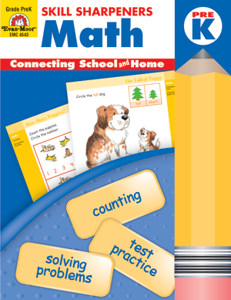# Skill Sharpeners Math, Pre-K

EMC4543
Regular price \$9.99 \$0.00

Skill Sharpeners Math, PreK contains 10 units that introduce the numbers 1-10. In each unit, one or more numbers is introduced followed by activity pages that practice beginning math skills. Each unit ends with an assessment page that reviews the math concepts introduced.

Beginning math skills practiced include:

• counting
• recognizing numbers
• patterning
• identifying geometric shapes
• comparing sizes
• recognizing most and least

Children also develop their fine motor skills by completing the fun activities.

Examples of the types of activities are:

• Trace and Write Numbers
• Cut and Paste
• Dot-to-Dot
• Count How Many
• Color the Shapes
• Circle the Ones That Are the Same
• Match